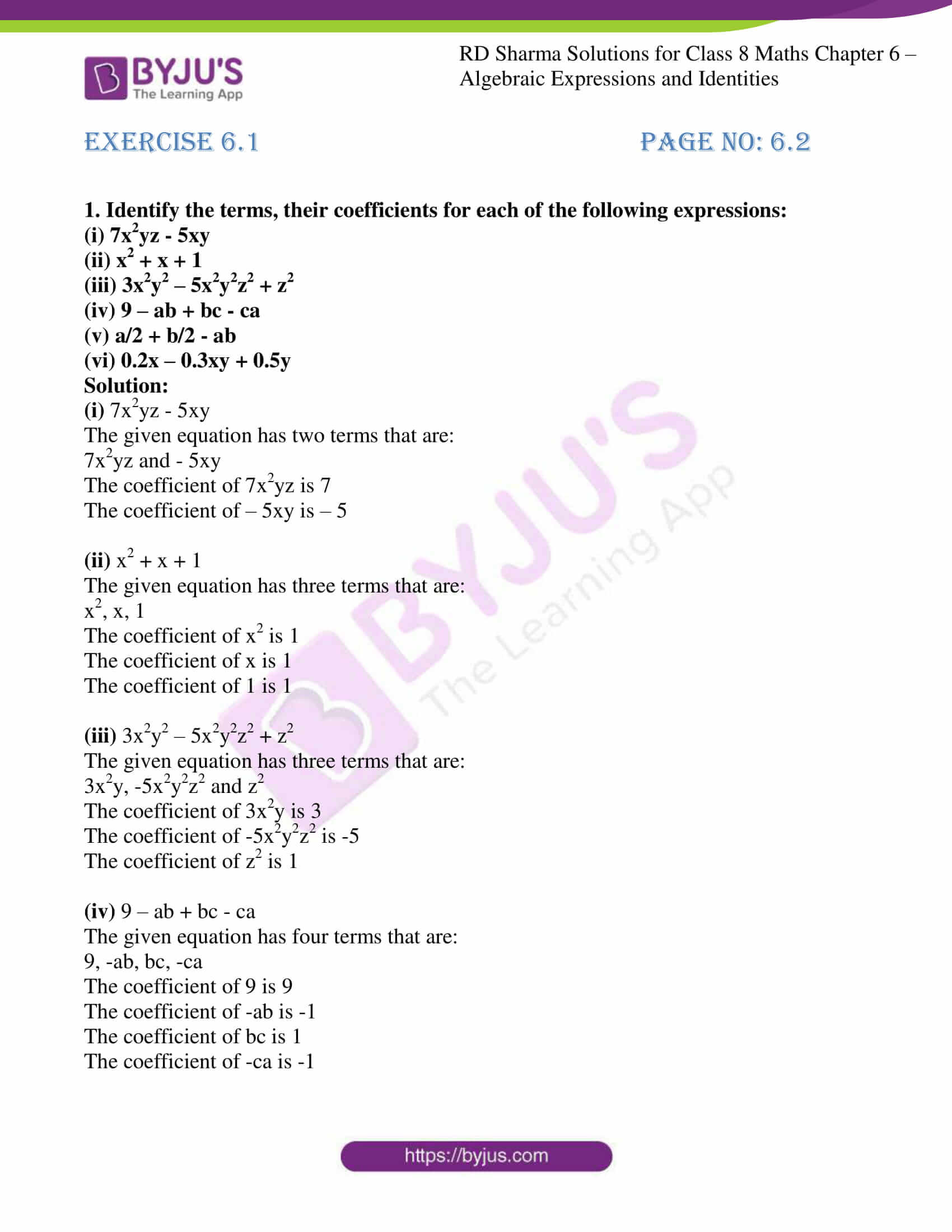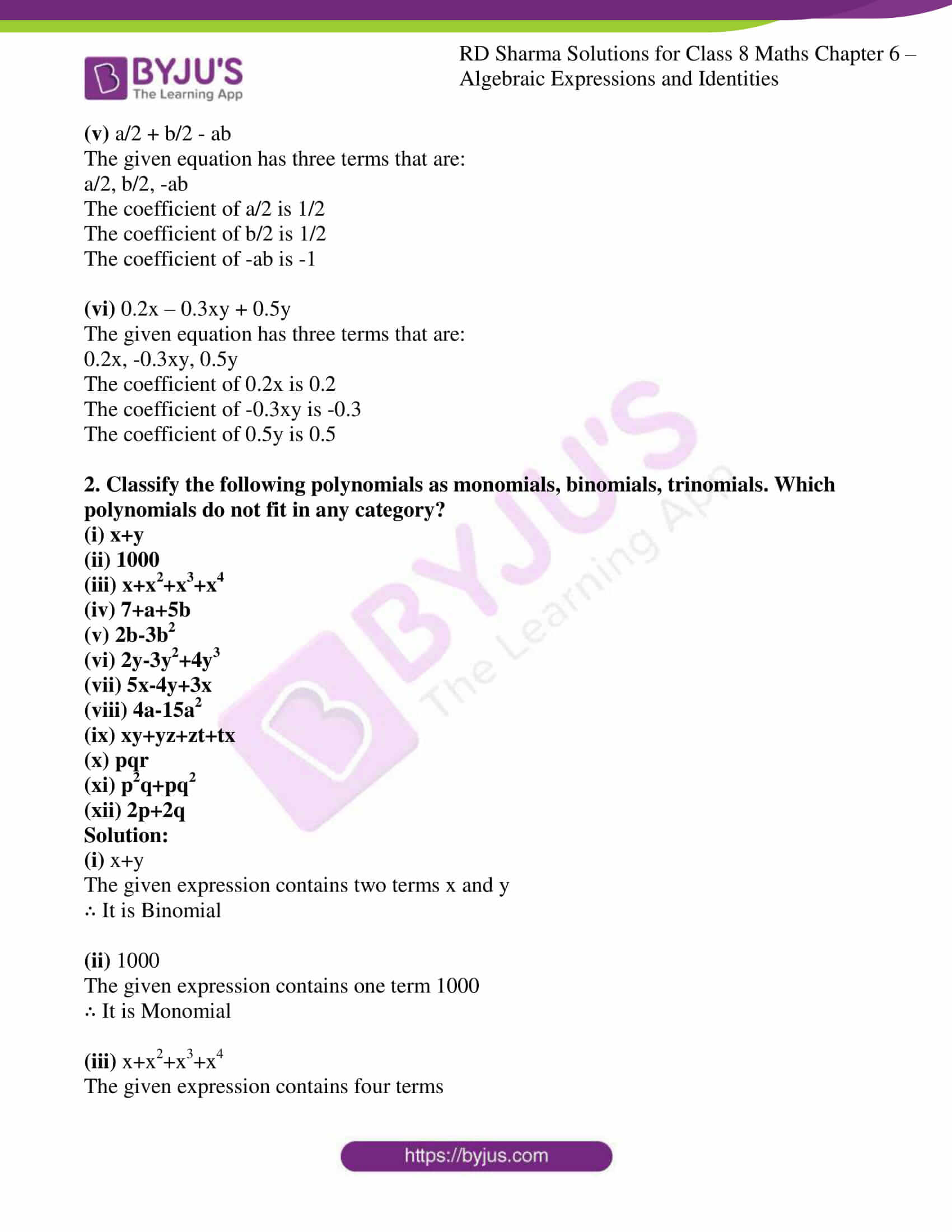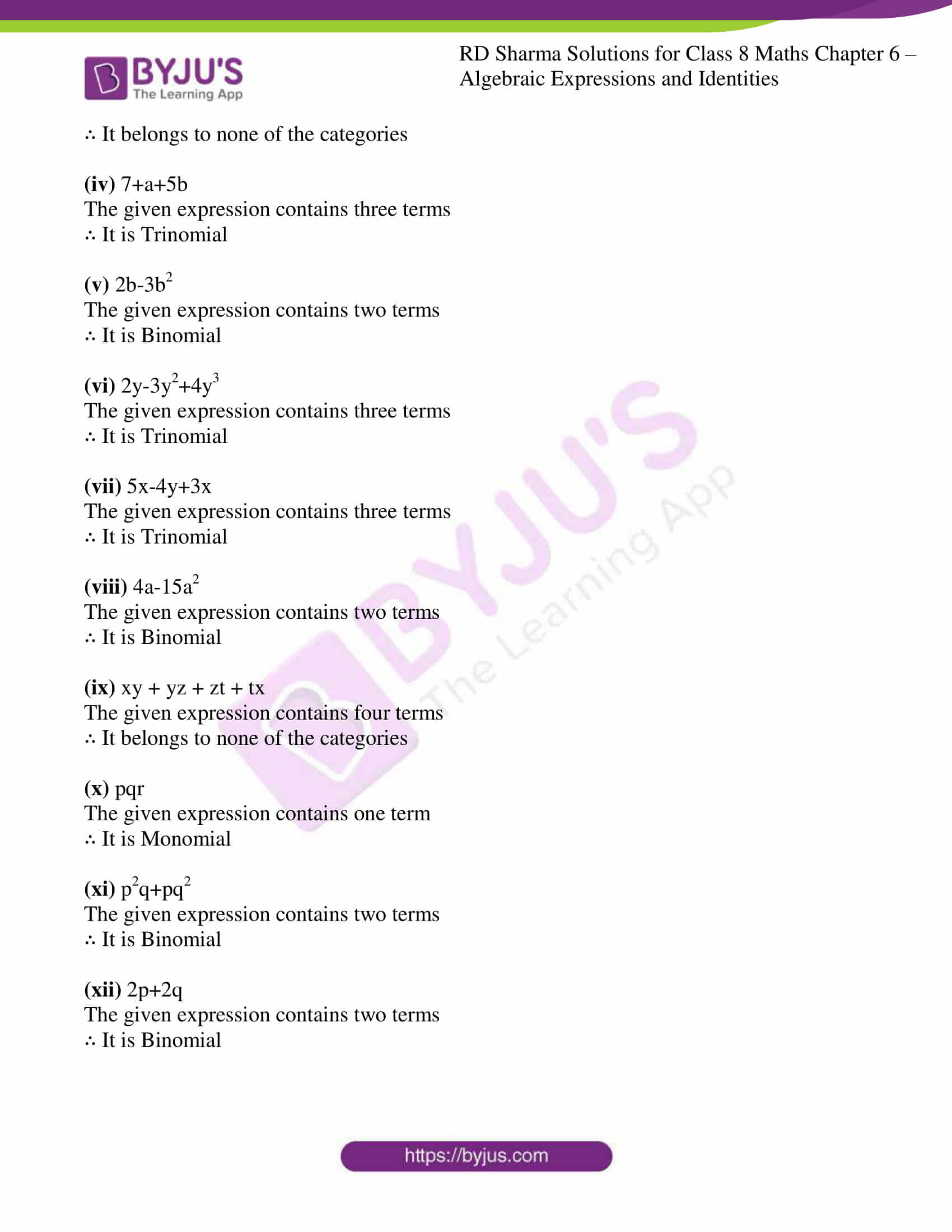# RD Sharma Solutions for Class 8 Maths Chapter 6 - Algebraic Expressions and Identities Exercise 6.1

Students can refer to pdf of RD Sharma Solutions Class 8 Maths which are designed by our expert team. RD Sharma Solutions is one of the best reference material for CBSE students. Learners can download the pdf from the links provided below. In this Exercise 6.1 of Chapter 6 algebraic expressions and identities, we shall discuss the basic definitions used in algebra like constants, variables, algebraic expressions, monomial, binomial, trinomial, factors and coefficients.

## Download the pdf of RD Sharma Solutions For Class 8 Maths Exercise 6.1 Chapter 6 Algebraic Expressions and Identities### Access Answers to RD Sharma Solutions for Class 8 Maths Exercise 6.1 Chapter 6 Algebraic Expressions and Identities

#### EXERCISE 6.1 PAGE NO: 6.2

1. Identify the terms, their coefficients for each of the following expressions:

(i) 7x2yz – 5xy

(ii) x2 + x + 1

(iii) 3x2y2 – 5x2y2z2 + z2

(iv) 9 – ab + bc – ca

(v) a/2 + b/2 – ab

(vi) 0.2x – 0.3xy + 0.5y

Solution:
(i)
7x2yz – 5xy

The given equation has two terms that are:

7x2yz and – 5xy

The coefficient of 7x2yz is 7

The coefficient of – 5xy is – 5

(ii) x2 + x + 1

The given equation has three terms that are:

x2, x, 1

The coefficient of x2 is 1

The coefficient of x is 1

The coefficient of 1 is 1

(iii) 3x2y2 – 5x2y2z2 + z2

The given equation has three terms that are:

3x2y, -5x2y2z2 and z2

The coefficient of 3x2y is 3

The coefficient of -5x2y2z2 is -5

The coefficient of z2 is 1

(iv) 9 – ab + bc – ca

The given equation has four terms that are:

9, -ab, bc, -ca

The coefficient of 9 is 9

The coefficient of -ab is -1

The coefficient of bc is 1

The coefficient of -ca is -1

(v) a/2 + b/2 – ab

The given equation has three terms that are:

a/2, b/2, -ab

The coefficient of a/2 is 1/2

The coefficient of b/2 is 1/2

The coefficient of -ab is -1

(vi) 0.2x – 0.3xy + 0.5y

The given equation has three terms that are:

0.2x, -0.3xy, 0.5y

The coefficient of 0.2x is 0.2

The coefficient of -0.3xy is -0.3

The coefficient of 0.5y is 0.5

2. Classify the following polynomials as monomials, binomials, trinomials. Which polynomials do not fit in any category?

(i) x+y
(ii) 1000
(iii) x+x2+x3+x4
(iv) 7+a+5b
(v) 2b-3b2
(vi) 2y-3y2+4y3
(vii) 5x-4y+3x
(viii) 4a-15a2
(ix) xy+yz+zt+tx
(x) pqr
(xi) p2q+pq2
(xii) 2p+2q

Solution:

(i) x+y

The given expression contains two terms x and y

∴ It is Binomial

(ii) 1000

The given expression contains one term 1000

∴ It is Monomial

(iii) x+x2+x3+x4

The given expression contains four terms

∴ It belongs to none of the categories

(iv) 7+a+5b

The given expression contains three terms

∴ It is Trinomial

(v) 2b-3b2

The given expression contains two terms

∴ It is Binomial

(vi) 2y-3y2+4y3

The given expression contains three terms

∴ It is Trinomial

(vii) 5x-4y+3x

The given expression contains three terms

∴ It is Trinomial

(viii) 4a-15a2

The given expression contains two terms

∴ It is Binomial

(ix) xy + yz + zt + tx

The given expression contains four terms

∴ It belongs to none of the categories

(x) pqr

The given expression contains one term

∴ It is Monomial

(xi) p2q+pq2

The given expression contains two terms

∴ It is Binomial

(xii) 2p+2q

The given expression contains two terms

∴ It is Binomial